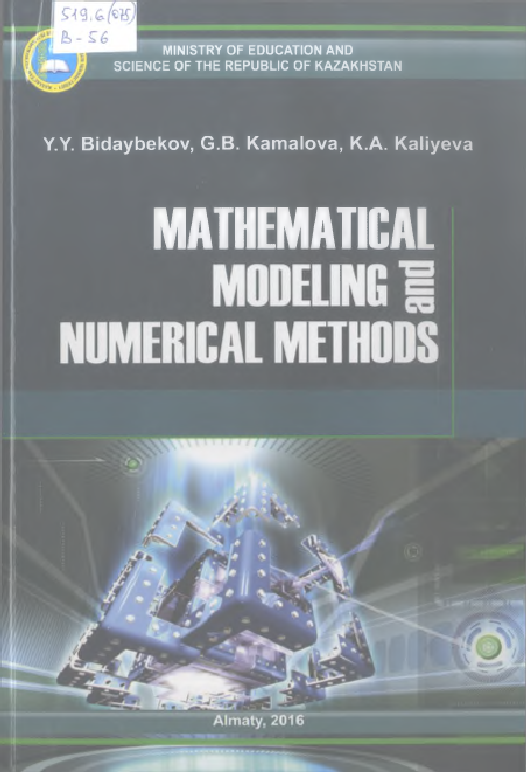#### Mathematical modeling and numerical methods: TextbookMathematical modeling and numerical methods: Textbook

Название: Mathematical modeling and numerical methods: Textbook Bidaybekov Y.Y. and etc. Книга English Bidaybekov Y.Y. and etc. Mathematical modeling and numerical methods: Textbook. - Almaty. 978-601-7529-94-9. Первый читальный зал mathematical, modeling, numerical methods This textbook is intended to provide particularly non-mathematicians with enough knowledge about the mathematical aspects of modeling such that they can deal at least with simple mathematical models on their own. Mathematical Modeling and Numerical Methods are addressed the three most important aspects in the field: mathematical models, computational methods and applications. The aim of this book is to provide an understanding of how Mathematical modeling associated with design, production, and operation of technical objects. This tutorial consists of three parts: I. Mathematical Modeling of physic-mechanical objects II. Introduction to MATLAB/Simulink software package III. Numerical Methods and Simulation Each part has been grouped into chapters. Every chapter is provided with examples that further elaborate on the text. This book is recommended for high schools’ students of physic-mathematical and computer science specialty and for all, who interested in mathematical modeling of the physic-mechanical processes and phenomenon.